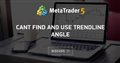# How to get the angle of a trendline and place a text object to it? - page 268

Although this was a long time ago, the above seemed to go around in circles, and didn't give a useful answer as far as I can figure. I noticed several other earlier threads that also provided no answer (https://www.forexfactory.com/showthread.php?t=428875 https://www.forexfactory.com/showthread.php?t=231003 ...). So for anyone else trying to get line/label angles right, this should help...

To get an angle that corresponds to the line angle on the price chart, you need to get the time and price co-ordinates into a common dimension. Since the chart is displayed on a screen, the chart's XY dimensions work great.

From the original code:

```         datetime time1 = (datetime)ObjectGetInteger(0, objName, OBJPROP_TIME1);
double price1 = ObjectGetDouble(0, objName, OBJPROP_PRICE1);
datetime time2 = (datetime)ObjectGetInteger(0, objName, OBJPROP_TIME2);
double price2 = ObjectGetDouble(0, objName, OBJPROP_PRICE2);
double angle_in_radians = MathArctan((price2-price1) / (time2-time1));
double angle_in_degree = 180 * angle_in_radians / MathArccos(-1.0);```

Rather than Arctan of the 2 different price and time dimensions as above, you would determine the arctan of the XY co-ordinates of the 2 price/time points:

int X2, Y2, X1, Y1;
ChartTimePriceToXY(0, 0, time2, price2, X2, Y2);
ChartTimePriceToXY(0, 0, time1, price1, X1, Y1);
angle_in_degrees = MathArctan( (double)(Y2-Y1)/(double)(X1-X2) )*180/M_PI;

And then set the angle of the label which will be the same as the line angle between the 2 price/time points:

ObjectSet(labelName, OBJPROP_ANGLE, angle_in_degrees);

However, if the line is moved or the XY dimensions change (zoom, autoscale, timeframe change etc...), these events need to be detected and the label angle recalculated and updated... Which unfortunately adds a considerable amount of GUI code for all the different events that can change the line angle.265

albry:

Although this was a long time ago, the above seemed to go around in circles, and didn't give a useful answer as far as I can figure. I noticed several other earlier threads that also provided no answer (https://www.forexfactory.com/showthread.php?t=428875 https://www.forexfactory.com/showthread.php?t=231003 ...). So for anyone else trying to get line/label angles right, this should help...

To get an angle that corresponds to the line angle on the price chart, you need to get the time and price co-ordinates into a common dimension. Since the chart is displayed on a screen, the chart's XY dimensions work great.

From the original code:

Rather than Arctan of the 2 different price and time dimensions as above, you would determine the arctan of the XY co-ordinates of the 2 price/time points:

int X2, Y2, X1, Y1;
ChartTimePriceToXY(0, 0, time2, price2, X2, Y2);
ChartTimePriceToXY(0, 0, time1, price1, X1, Y1);
angle_in_degrees = MathArctan( (double)(Y2-Y1)/(double)(X1-X2) )*180/M_PI;

And then set the angle of the label which will be the same as the line angle between the 2 price/time points:

ObjectSet(labelName, OBJPROP_ANGLE, angle_in_degrees);

However, if the line is moved or the XY dimensions change (zoom, autoscale, timeframe change etc...), these events need to be detected and the label angle recalculated and updated... Which unfortunately adds a considerable amount of GUI code for all the different events that can change the line angle.

You did correctly, you simple needed to multiply by the x/y ratio at the end and receive a non-changing angle.

You can look at the code I published here: https://www.mql5.com/en/forum/155802/page2#comment_7391292Cant find and use trendline angle
• 2018.05.10
• www.mql5.com
What I want to do is to create a trendline with angle based on 2 anchor points and then find the angle using ObjectGetDouble() so it can help me do...